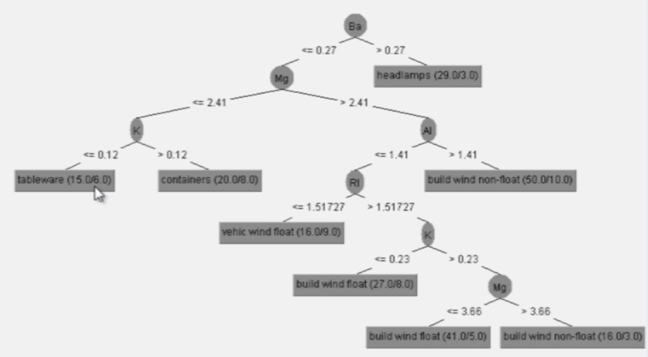## Want to keep learning?

This content is taken from the The University of Waikato's online course, Data Mining with Weka. Join the course to learn more.
1.20

# How would you apply this in real life?

So, you can use Weka to build a classifier. So what?

In the video I showed you how to use Weka to build a J48 classifier for the glass.arff dataset, and look at the evaluation results – the percentage accuracy – and the tree produced by J48. In the quiz you built J48 classifiers for that dataset and another, labor.arff, and looked at the confusion matrix and percentage of correctly classified instances.

But the real goal is to use the classifier on new data. How do you do that? Well, you could imagine following down the decision tree that I showed in the video manually, with a new instance. (To aid readability I’ve made this tree slightly smaller than the one in the video by specifying minNumObj=15):You know the values of the attributes but not the class, and you want to find it. First look at the Barium (Ba) content. If it’s large ($$>0.27$$), then the class must be headlamps. If it’s small ($$\leq 0.27$$), then look at Magnesium (Mg). If that’s small ($$\leq 2.41$$), then look at potassium (K), and if that’s small ($$\leq 0.12$$), then we’ve got tableware.

Suppose you had these two instances:

RI Na Mg Al Si K Ca Ba Fe
1.5 14.2 0 2.8 73.5 0 9.0 0.4 0.09
1.5 14.1 2.2 1.7 72.7 0 9.3 0 0

The first one has Ba = 0.4, which is $$>0.27$$, so this instance must be headlamps. For the second, Ba = 0, which is $$\leq 0.27$$; Mg = 2.2, which is $$\leq 2.41$$; and K = 0, which is $$\leq 0.12$$; so this instance must be tableware.

But there’s no need to do this manually! Weka can apply any classifier to a test file of new instances, or save the classifier for future use. It’s all very easy, and you’ll learn how to do such things later in the course.

Remember, this week is a whirlwind tour, and consequently superficial. You’re going to learn much more about how to build, evaluate, and apply classifiers in subsequent weeks. But, for now, let’s press on with our tour.